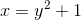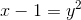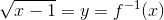# High School Math : Understanding Inverse Functions

## Example Questions

### Example Question #1 : Understanding Inverse Functions

Let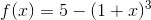. What is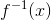?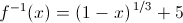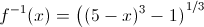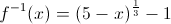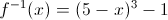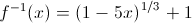Explanation:

We are asked to find, which is the inverse of a function.

In order to find the inverse, the first thing we want to do is replace f(x) with y. (This usually makes it easier to separate x from its function.).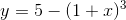Next, we will swap x and y.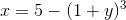Then, we will solve for y. The expression that we determine will be equal to.Subtract 5 from both sides.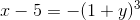Multiply both sides by -1.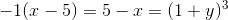We need to raise both sides of the equation to the 1/3 power in order to remove the exponent on the right side.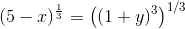We will apply the general property of exponents which states that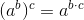.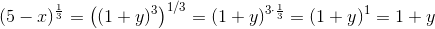Laslty, we will subtract one from both sides.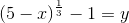The expression equal to y is equal to the inverse of the original function f(x). Thus, we can replace y with.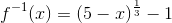The answer is.

### Example Question #2 : Understanding Inverse Functions

What is the inverse of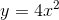?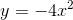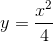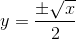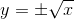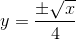Explanation:

The inverse ofrequires us to interchangeandand then solve for.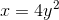Then solve for:### Example Question #371 : Algebra Ii

If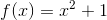, what is?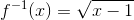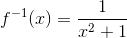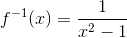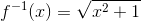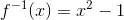Explanation:

To find the inverse of a function, exchange theandvariables and then solve for.